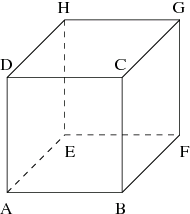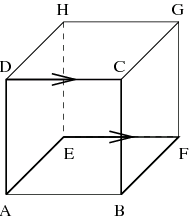시간 제한 메모리 제한 제출 정답 맞은 사람 정답 비율
1 초 128 MB 0 0 0 0.000%

## 문제

A Byteotian ant is walking along the edges of ABCDEFGH cube:It tries to find out, in how many ways it can go from one given vertex, to another given vertex, walking along exactly k edges (when the ant enters an edge, it will not turn back and will finally reach the second end of this edge). If the ant goes through some edge x times, we count this edge x times. The ant would like to have interesting routes, that is if the ant is in some vertex, it would like to leave this vertex using an edge other than the edge recently used to enter this vertex (i.e. it want not to use the same edge twice in a row).

Our ant is not so smart, because it can only count using integers from 0 to p - 1, for some p, so you should compute the result modulo p.

Write a program which:

• reads the starting and the ending vertex of the ant's route, number of edges on the ant's route, and integer p,
• computes number of interesting routes, which satisfy the ant's requests, modulo p,
• writes the answer to the standard output.

## 입력

The first line of the standard input contains two capital English letters v1 and v2 (Av1, v2H, v1v2), separated by a single space. The two letters denote the starting and ending vertex of the ant's route respectively. The second line contains two integers k and p (1 ≤ k ≤ 2 000 000 000, 2 ≤ p ≤ 1 000 000 000), separated by a single space.

## 출력

Exactly one integer is to be written on the standard output. This integer is the number of interesting routes from the vertex v1 to the vertex v2, containing exactly k edges, modulo p.

## 예제 입력 1

A B
3 100


## 예제 출력 1

2


## 힌트